Question

# An ideal gaseous reaction occurs at a constant pressure of 35.0 atm and releases 55.8 kJ...

An ideal gaseous reaction occurs at a constant pressure of 35.0 atm and releases 55.8 kJ of heat. Before the reaction, the volume of the system was 8.20 L. After the reaction, the volume of the system was 3.01 L. Calculate the total change in internal energy for the system.

From first law of thermodynamics , we know that

dU = dq + dW

Here, dU is the change in internal Energy

dq is the amount of Heat exchange

dW is the amount of Work done

According to the IUPAC Conversation , Heat released by the system is regarded as the negative quantity .

Hence ,

dq= -55.8kJ = -55800 J

Work done against constant pressure .

We know that

dW= - P dV

dW =-P ( V​​​​​​2 - V​​​​​​1 )

Here , P = 35.0 atm , V​​​​​​2 = 3.01 L & V​​​​​1 = 8.20 L

dW = - 35.0 atm ( 3.01- 8.20) L

dW = 181.65 L atm

1 L atm = 101.325 J

Hence we get

dW = 181.65 × 101.325 J

dW = 18405.686 J

Hence internal energy

dU = dq + dW

= -55800 J + 18405.686 J

= - 37394.31 J

= -37.39431 KJ

Hence total Change in internal energy = -37.39431 KJ

#### Earn Coins

Coins can be redeemed for fabulous gifts.

Similar Homework Help Questions
• ### DIFCIU idue Part B A gaseous reaction occurs at a constant pressure of 35.0 atm and...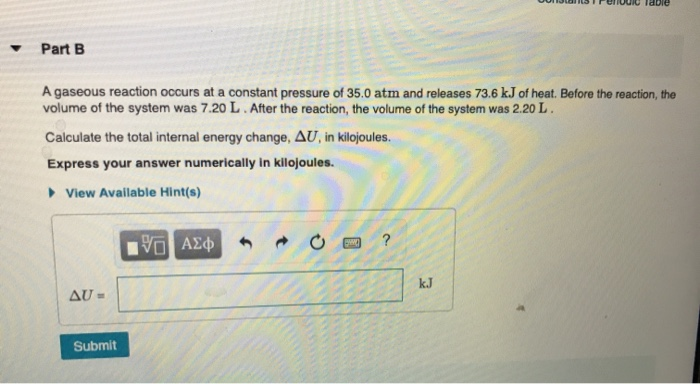DIFCIU idue Part B A gaseous reaction occurs at a constant pressure of 35.0 atm and releases 73.6 kJ of heat. Before the reaction, the volume of the system was 7.20 L. After the reaction, the volume of the system was 2.20 L. Calculate the total internal energy change, AU, in kilojoules. Express your answer numerically in kilojoules. View Available Hint(s) YO AE AU- Submit

• ### An ideal gaseous reaction (which is a hypothetical gaseous reaction that conforms to the laws governing...

An ideal gaseous reaction (which is a hypothetical gaseous reaction that conforms to the laws governing gas behavior) occurs at a constant pressure of 50.0 atm and releases 62.9 kJ of heat. Before the reaction, the volume of the system was 6.60 L . After the reaction, the volume of the system was 3.00 L . Calculate the total internal energy change, ΔU, in kilojoules.

• ### An ideal gaseous reaction (which is a hypothetical gaseous reaction that conforms to the laws governing...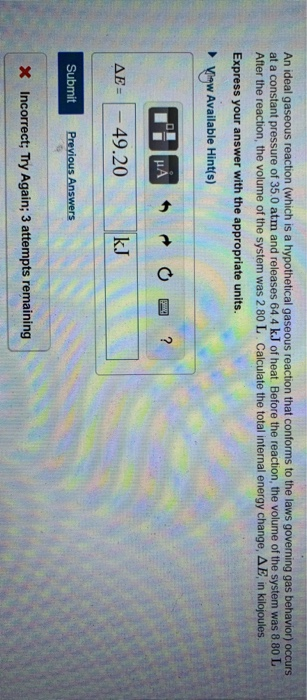An ideal gaseous reaction (which is a hypothetical gaseous reaction that conforms to the laws governing gas behavior) occurs at a constant pressure of 35.0 atm and releases 64.4 kJ of heat. Before the reaction, the volume of the system was 8.80 L After the reaction, the volume of the system was 2.80 L. Calculate the total internal energy change, AE, in kilojoules. Express your answer with the appropriate units. Vigw Available Hint(s) AE = - 49.20 kJ Submit Previous...

• ### An ideal gaseous reaction (which is a hypothetical gaseous reaction that conforms to the laws governing gas behavior) oc...

An ideal gaseous reaction (which is a hypothetical gaseous reaction that conforms to the laws governing gas behavior) occurs at a constant pressure of 50.0 atm and releases 65.0 kJ of heat. Before the reaction, the volume of the system was 7.60 L . After the reaction, the volume of the system was 2.20 L . Calculate the total internal energy change, ΔE, in kilojoules. Express your answer with the appropriate units.

• ### An ideal gaseous reaction (which is a hypothetical gaseous reaction that conforms to the laws governing...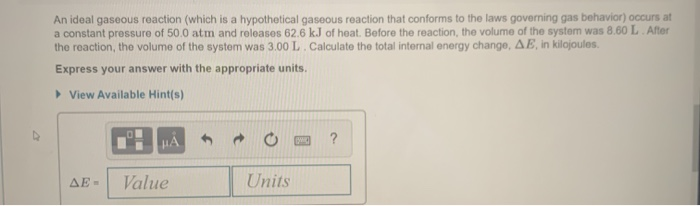An ideal gaseous reaction (which is a hypothetical gaseous reaction that conforms to the laws governing gas behavior) occurs at a constant pressure of 50.0 atm and releases 62.6 kJ of heat. Before the reaction, the volume of the system was 8.60 L After the reaction, the volume of the system was 3.00 L. Calculate the total internal energy change, AE, in kilojoules. Express your answer with the appropriate units. View Available Hint(s) HAO? AE - Value Units

• ### Part B An ideal gaseous reaction (which is a hypothetical gaseous reaction that conforms to the...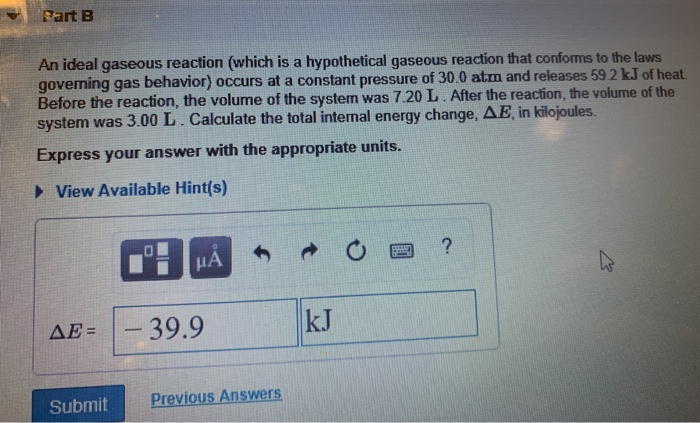Part B An ideal gaseous reaction (which is a hypothetical gaseous reaction that conforms to the laws governing gas behavior) occurs at a constant pressure of 30.0 atm and releases 59.2 kJ of heat. Before the reaction, the volume of the system was 7.20 L. After the reaction, the volume of the system was 3.00 L. Calculate the total internal energy change, AE in kilojoules. Express your answer with the appropriate units. View Available Hint(s) 1: HÅR O a ?...

• ### Part B An ideal gaseous reaction (which is a hypothetical gaseous reaction that conforms to the...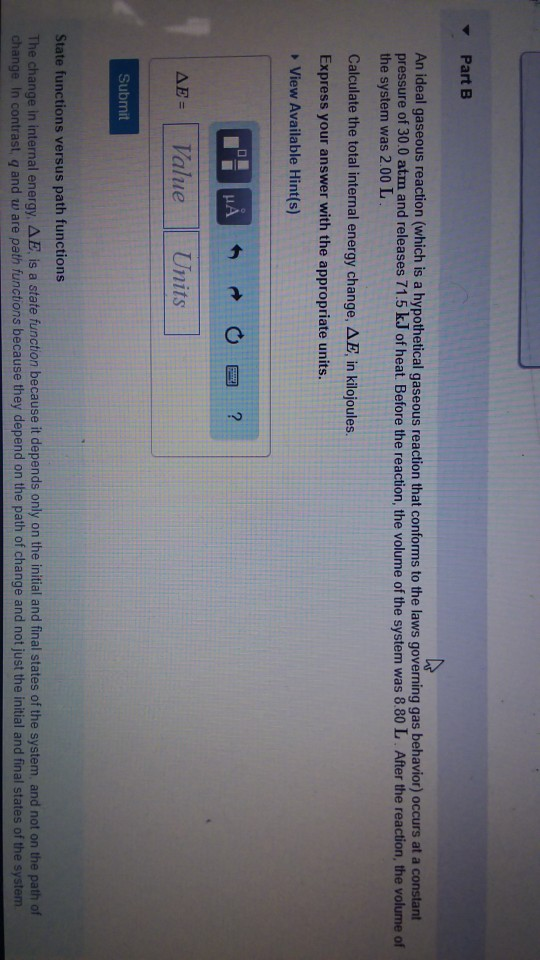Part B An ideal gaseous reaction (which is a hypothetical gaseous reaction that conforms to the laws governing gas behavior) occurs at a constart pressure of 30.0 atm and releases 71.5 k.J of heat. Before the reaction, the volume of the system was 8.80 L After the reaction, the volume of the system was 2.00 L Calculate the total internal energy change, ??, in kilojoules. Express your answer with the appropriate units. View Available Hint(s) ??-( Value Units Submit State...

• ### The First Law of Thermodynamics

A gaseous reaction occurs at a constant pressure of 50.0 atm and releases 64.0 kJ of heat. Before the reaction, the volume of the system was 6.60 L. After thereaction, the volume of the system was 3.00 L. Calculate the total internal energy change, Delta U, in kilojoules.

• ### A mole of X reacts at a constant pressure of 43.0 atm via the reaction X(g)+4Y(g)→2Z(g), ΔH∘=−75.0 kJ Before the reacti...

A mole of X reacts at a constant pressure of 43.0 atm via the reaction X(g)+4Y(g)→2Z(g), ΔH∘=−75.0 kJ Before the reaction, the volume of the gaseous mixture was 5.00 L. After the reaction, the volume was 2.00 L. Calculate the value of the total energy change, ΔE, in kilojoules.

• ### A mole of X reacts at a constant pressure of 43.0 atm via the reaction X(g)+4Y(g)→2Z(g),    &nbs...

A mole of X reacts at a constant pressure of 43.0 atm via the reaction X(g)+4Y(g)→2Z(g),      ΔH∘=−75.0 kJ Before the reaction, the volume of the gaseous mixture was 5.00 L. After the reaction, the volume was 2.00 L. Calculate the value of the total energy change, ΔE, in kilojoules. Express your answer numerically in kilojoules.